The SEVERITY Procedure

Statistics of Fit

Subsections:

PROC SEVERITY computes and reports various statistics of fit to indicate how well the estimated model fits the data. The statistics belong to two categories: likelihood-based statistics and EDF-based statistics. Neg2LogLike, AIC, AICC, and BIC are likelihood-based statistics, and KS, AD, and CvM are EDF-based statistics. The following subsections provide definitions of each.

Likelihood-Based Statistics of Fit

Let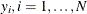, denote the response variable values. Let L be the likelihood as defined in the section Likelihood Function. Let p denote the number of model parameters that are estimated. Note that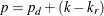, where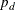is the number of distribution parameters, k is the number of all regression parameters, and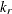is the number of regression parameters that are found to be linearly dependent (redundant) on other regression parameters. Given this notation, the likelihood-based statistics are defined as follows:

Neg2LogLike

The log likelihood is reported as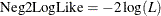The multiplying factor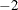makes it easy to compare it to the other likelihood-based statistics. A model that has a smaller value of Neg2LogLike is deemed better.

AIC

Akaike’s information criterion (AIC) is defined as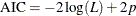A model that has a smaller AIC value is deemed better.

AICC

The corrected Akaike’s information criterion (AICC) is defined as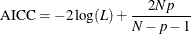A model that has a smaller AICC value is deemed better. It corrects the finite-sample bias that AIC has when N is small compared to p. AICC is related to AIC as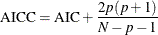As N becomes large compared to p, AICC converges to AIC. AICC is usually recommended over AIC as a model selection criterion.

BIC

The Schwarz Bayesian information criterion (BIC) is defined as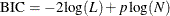A model that has a smaller BIC value is deemed better.

EDF-Based Statistics

This class of statistics is based on the difference between the estimate of the cumulative distribution function (CDF) and the estimate of the empirical distribution function (EDF). A model that has a smaller value of the chosen EDF-based statistic is deemed better.

Letdenote the sample of N values of the response variable. Let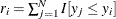denote the number of observations with a value less than or equal to, where I is an indicator function. Let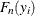denote the EDF estimate that is computed by using the method that you specify in the EMPIRICALCDF= option. Let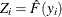denote the estimate of the CDF. Let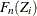denote the EDF estimate of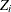values that are computed using the same method that is used to compute the EDF ofvalues. Using the probability integral transformation, if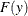is the true distribution of the random variable Y, then the random variable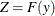is uniformly distributed between 0 and 1 (D’Agostino and Stephens, 1986, Ch. 4). Thus, comparingwith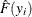is equivalent to comparingwith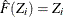(uniform distribution).

Note the following two points regarding which CDF estimates are used for computing the test statistics:

• If you specify regression effects, then the CDF estimatesthat are used for computing the EDF test statistics are from a mixture distribution. See the section CDF and PDF Estimates with Regression Effects for more information.

• If the EDF estimates are conditional because of the truncation information, then each unconditional estimateis converted to a conditional estimate using the method described in the section Truncation and Conditional CDF Estimates.

In the following, it is assumed thatdenotes an appropriate estimate of the CDF if you specify any truncation or regression effects. Given this, the EDF-based statistics of fit are defined as follows:

KS

The Kolmogorov-Smirnov (KS) statistic computes the largest vertical distance between the CDF and the EDF. It is formally defined as follows: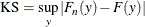If the STANDARD method is used to compute the EDF, then the following formula is used: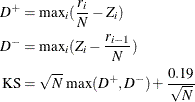Note that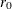is assumed to be 0.

If the method used to compute the EDF is any method other than the STANDARD method, then the following formula is used: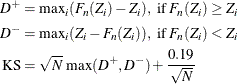The Anderson-Darling (AD) statistic is a quadratic EDF statistic that is proportional to the expected value of the weighted squared difference between the EDF and CDF. It is formally defined as follows: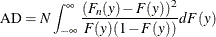If the STANDARD method is used to compute the EDF, then the following formula is used: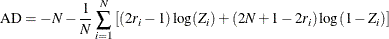If the method used to compute the EDF is any method other than the STANDARD method, then the statistic can be computed by using the following two pieces of information:

• If the EDF estimates are computed using the KAPLANMEIER or MODIFIEDKM methods, then EDF is a step function such that the estimate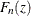is a constant equal to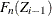in interval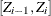. If the EDF estimates are computed using the TURNBULL method, then there are two types of intervals: one in which the EDF curve is constant and the other in which the EDF curve is theoretically undefined. For computational purposes, it is assumed that the EDF curve is linear for the latter type of the interval. For each method, the EDF estimate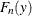at y can be written as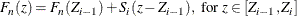where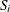is the slope of the line defined as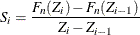For the KAPLANMEIER or MODIFIEDKM method,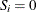in each interval.

• Using the probability integral transform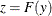, the formula simplifies to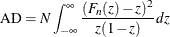The computation formula can then be derived from the following approximation: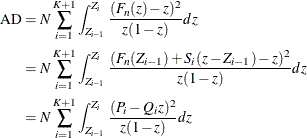where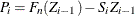,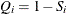, and K is the number of points at which the EDF estimate are computed. For the TURNBULL method,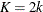for some k.

Assuming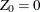,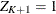,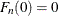, and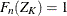yields the following computation formula: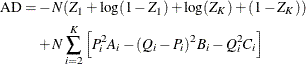where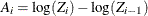,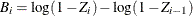, and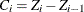.

If EDF estimates are computed using the KAPLANMEIER or MODIFIEDKM method, then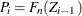and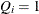, which simplifies the formula as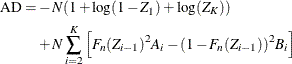CvM

The Cramér-von Mises (CvM) statistic is a quadratic EDF statistic that is proportional to the expected value of the squared difference between the EDF and CDF. It is formally defined as follows: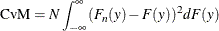If the STANDARD method is used to compute the EDF, then the following formula is used: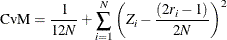If the method used to compute the EDF is any method other than the STANDARD method, then the statistic can be computed by using the following two pieces of information:

• As described previously for the AD statistic, the EDF estimates are assumed to be piecewise linear such that the estimateat y iswhereis the slope of the line defined asFor the KAPLANMEIER or MODIFIEDKM method,in each interval.

• Using the probability integral transform, the formula simplifies to: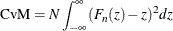The computation formula can then be derived from the following approximation: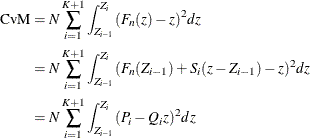where,, and K is the number of points at which the EDF estimate are computed. For the TURNBULL method,for some k.

Assuming,, andyields the following computation formula: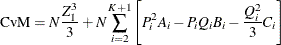where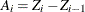,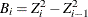, and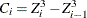.

If EDF estimates are computed using the KAPLANMEIER or MODIFIEDKM method, thenand, which simplifies the formula as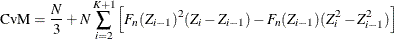which is similar to the formula proposed by Koziol and Green (1976).